Next: 1-d problem with Dirichlet Up: Poisson's equation Previous: Poisson's equation

## Introduction

In this section, we shall discuss some simple numerical techniques for solving Poisson's equation: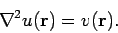(108)

Here,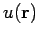is usually some sort of potential, whereas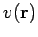is a source term. The solution to the above equation is generally required in some simply-connected volumebounded by a closed surface. There are two main types of boundary conditions to Poisson's equation. In so-called Dirichlet boundary conditions, the potential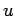is specified on the bounding surface. In so-called Neumann boundary conditions, the normal gradient of the potential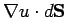is specified on the bounding surface.

Poisson's equation is of particular importance in electrostatics and Newtonian gravity. In electrostatics, we can write the electric field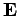in terms of an electric potential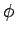: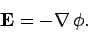(109)

The potential itself satisfies Poisson's equation: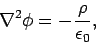(110)

where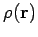is the charge density, and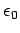the permittivity of free-space. In Newtonian gravity, we can write the force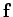exerted on a unit test mass in terms of a gravitational potential: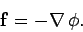(111)

The potential satisfies Poisson's equation: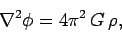(112)

whereis the mass density, and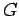the universal gravitational constant.Next: 1-d problem with Dirichlet Up: Poisson's equation Previous: Poisson's equation
Richard Fitzpatrick 2006-03-29